HashMap学习笔记

HashMap采用数组+链表的数据结构，只是在jdk1.7和1.8的实现上有所不同，下面，简单的分析一下，方便自己更加深刻的理解这种典型的key-value的数据结构。

1.1.jdk1.7实现原理简单分析

1.7的HashMap数据结构图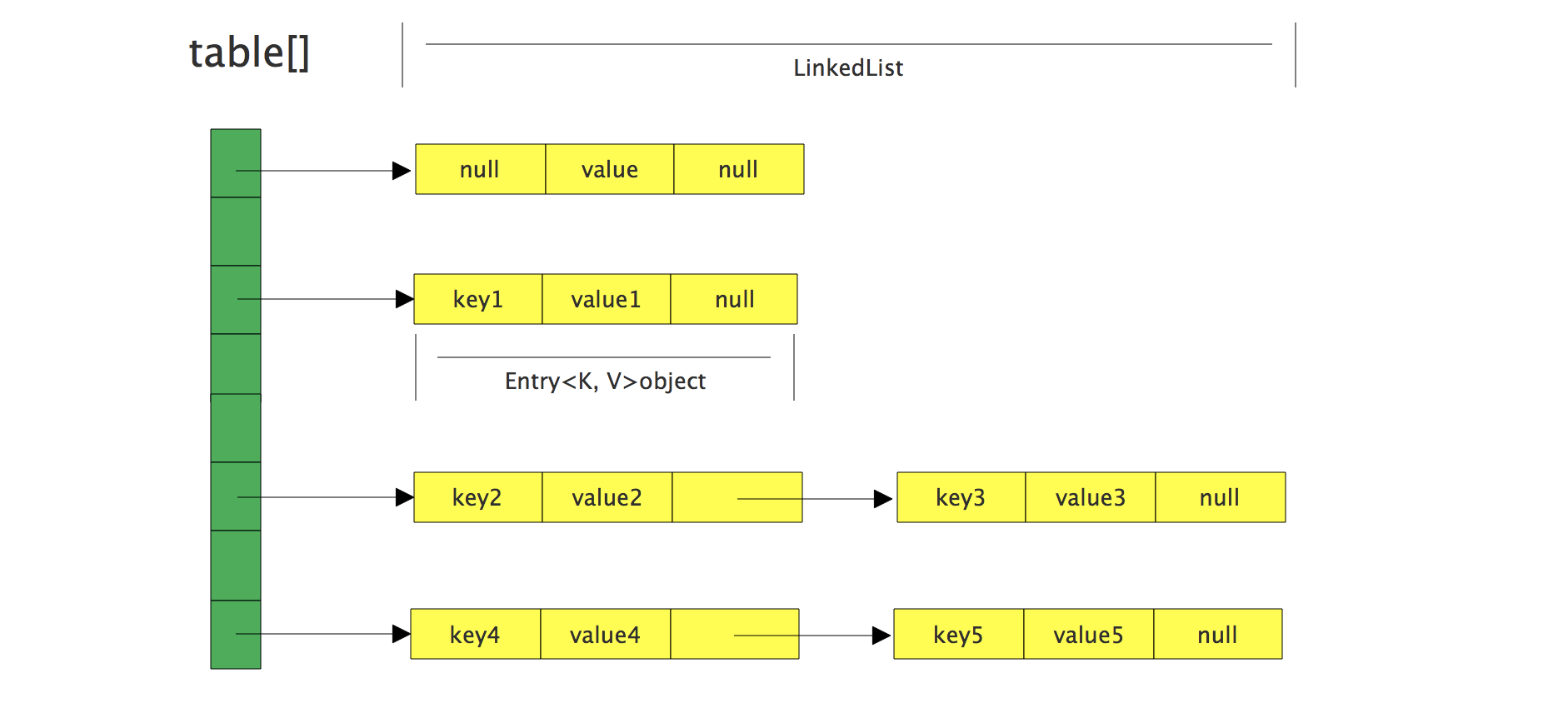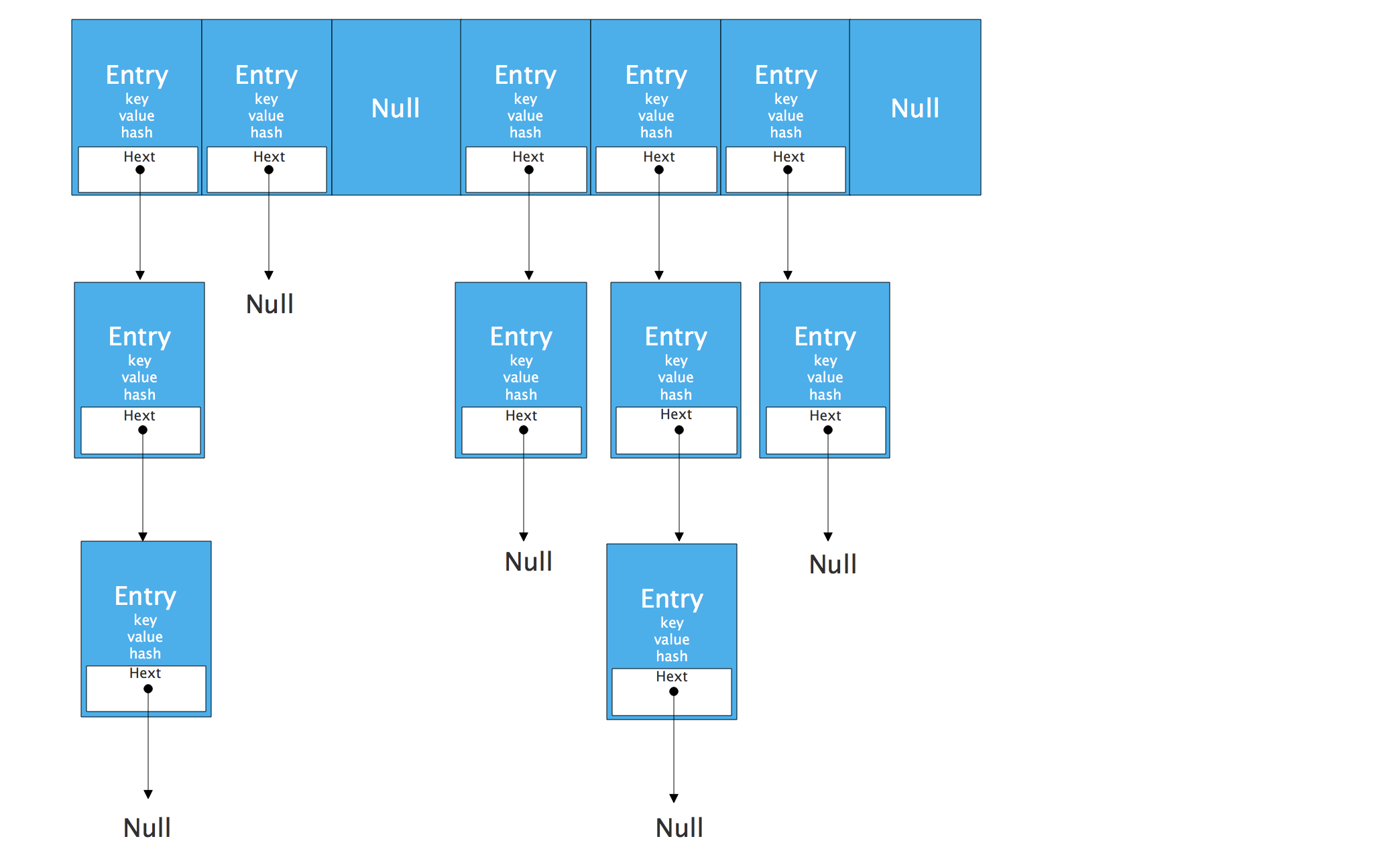HashMap 通过 key 的 hashCode 经过扰动函数处理过后得到 hash 值，然后通过 (n - 1) & hash 判断当前元素存放的位置（这里的 n 指的是数组的长度），如果当前位置存在元素的话，就判断该元素与要存入的元素的 hash 值以及 key 是否相同，如果相同的话，直接覆盖，不相同就通过拉链法解决冲突。

1.7的HashMap类中的常量

/** 初始化桶大小，HashMap底层是数组，这个是数组默认的大小 */
static final int DEFAULT_INITIAL_CAPACITY = 1 << 4; // aka 16

/**  桶的最大值 */
static final int MAXIMUM_CAPACITY = 1 << 30;

/** 默认的负载因子 */
static final float DEFAULT_LOAD_FACTOR = 0.75f;

static final Entry<?,?>[] EMPTY_TABLE = {};

/** table真正存放数据的数组 */
transient Entry<K,V>[] table = (Entry<K,V>[]) EMPTY_TABLE;

/** map中存放数据的大小 */
transient int size;

/** 桶大小。可以在初始化的时候显示指定 */
int threshold;

/** 负载因子，可以在初始化的时候显示指定 */

threshold桶大小

threshold桶大小，也叫临界值，threshold = capacity \* loadFactor当HashMap的Size>=threshold的时候，那么就要考虑对数组的扩增了，也就是说，这个的意思就是 threshold是衡量数组是否需要扩增的一个标准

table存放数据的数组

table数组中存放的是Entry类型的数据，下面我们简单看看Entry的定义。

static class Entry<K,V> implements Map.Entry<K,V> {
final K key;
V value;
Entry<K,V> next;
int hash;
/** 创建一个新的Entry */
Entry(int h, K k, V v, Entry<K,V> n) {
value = v;
next = n;
key = k;
hash = h;
}
}

Entry是一个内部类，其中的key就是写入的键，value就是写入的值，由于HashMap由数组+链表的形式，这里的next就是用于实现链表结构。hash存放的事当前key的hashcode值。

put()方法

public V put(K key, V value) {
/** 判断当前数组是否需要初始化 */
if (table == EMPTY_TABLE) {
inflateTable(threshold);
}
/** 如果key为空，则put一个空值进去 */
if (key == null)
return putForNullKey(value);
/** 根据key计算出hashcode值 */
int hash = hash(key);
/** 根据计算的hashcode值定位所在的桶 */
int i = indexFor(hash, table.length);
/** 如果桶是一个链表则需要遍历判断里面的 hashcode、key 是否和传入 key 相等， */
for (Entry<K,V> e = table[i]; e != null; e = e.next) {
Object k;
/** 如果相等则进行覆盖，并返回原来的值 */
if (e.hash == hash && ((k = e.key) == key || key.equals(k))) {
V oldValue = e.value;
e.value = value;
e.recordAccess(this);
return oldValue;
}
}
modCount++;
/** 如果桶是空的，说明当前位置没有数据存入；新增一个 Entry 对象写入当前位置 */
return null;
}

新增一个Entry

void addEntry(int hash, K key, V value, int bucketIndex) {
/** 判断当前HashMap的size与临界值的大小，判断是否需要扩容操作 */
if ((size >= threshold) && (null != table[bucketIndex])) {
/** 如果需要扩容，就进行2倍扩容 */
resize(2 * table.length);
/** 将当前的key重新hash并定位 */
hash = (null != key) ? hash(key) : 0;
bucketIndex = indexFor(hash, table.length);
}
/** 创建一个Entry,如果当前桶存在元素，就形成链表 */
createEntry(hash, key, value, bucketIndex);
}
void createEntry(int hash, K key, V value, int bucketIndex) {
Entry<K,V> e = table[bucketIndex];
table[bucketIndex] = new Entry<>(hash, key, value, e);
size++;
}

put()方法简单将如下：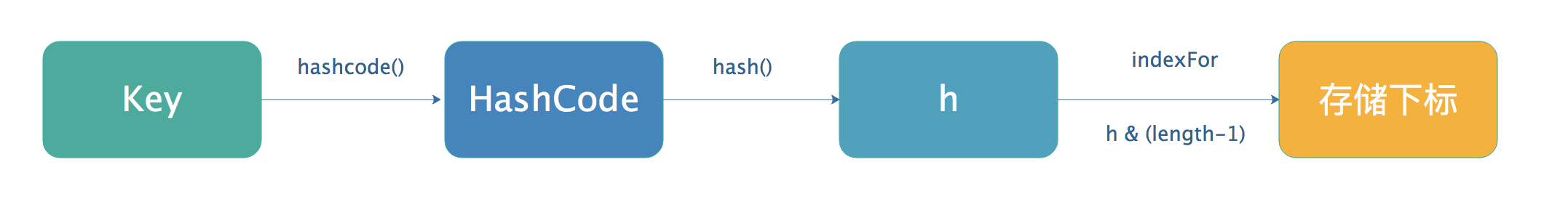get()方法

public V get(Object key) {
/** 如果key为null,就去数组的位置找 */
if (key == null)
return getForNullKey();
/** 根据key获取Entry */
Entry<K,V> entry = getEntry(key);
return null == entry ? null : entry.getValue();
}
final Entry<K,V> getEntry(Object key) {
/** 如果当前HashMap的size都为0，那就直接返回null */
if (size == 0) {
return null;
}
/** 根据key计算hashcode值,然后定位到具体的桶中 */
int hash = (key == null) ? 0 : hash(key);
/** 判断是否是链表，为链表则需要遍历直到 key 及 hashcode 相等时候就返回值*/
for (Entry<K,V> e = table[indexFor(hash, table.length)];
e != null;
e = e.next) {
Object k;
/** 不是链表就根据 key、key 的 hashcode 是否相等来返回值*/
if (e.hash == hash &&
((k = e.key) == key || (key != null && key.equals(k))))
return e;
}
/** 啥都没取到就直接返回 null */
return null;
}

1.2.jdk1.8实现原理简单分析

**数组：**数据存储是连续的，占用内存很大，所以空间复杂度较高，但是二分查找的时间复杂度为O(1)，简单讲就是，数组寻址容易，插入和删除较为困难

**链表：**存储区间零散，所以内存较为宽松，故空间复杂度较低，但是时间复杂的高，为O(n)，简单讲就是，链表寻址困难，插入和删除较为容易

1.8的HashMap的数据结构图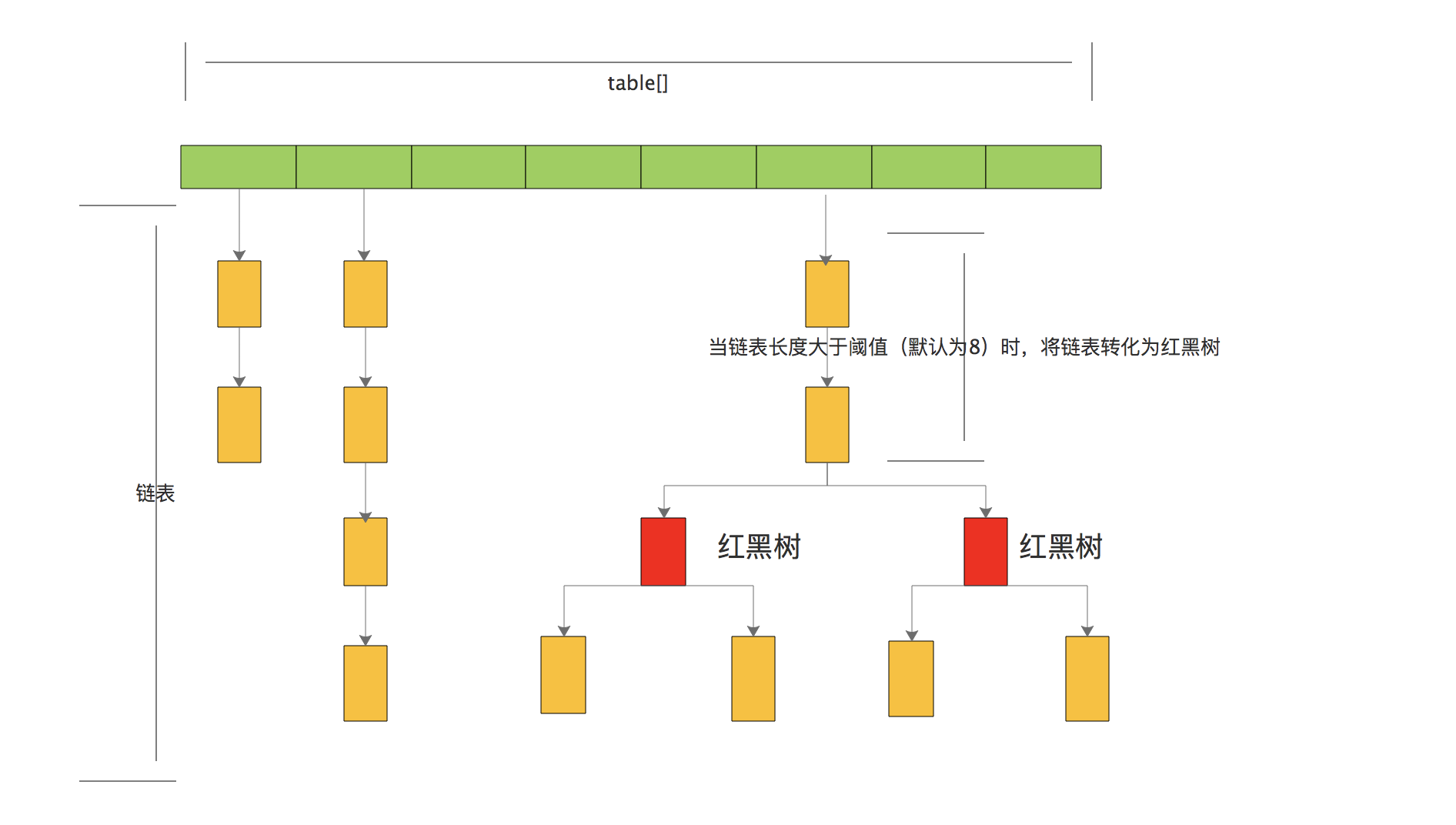1.8的HashMap类的常量

/** 默认的初始容量16 */
static final int DEFAULT_INITIAL_CAPACITY = 1 << 4; // aka 16

/** 最大的容量 */
static final int MAXIMUM_CAPACITY = 1 << 30;

/** 默认的填充因子 */
static final float DEFAULT_LOAD_FACTOR = 0.75f;

/** 当桶上的节点数量大于8时，会将链表转为红黑树 */
static final int TREEIFY_THRESHOLD = 8;

/** 当桶上的节点数量小于6时，会将红黑树转为链表 */
static final int UNTREEIFY_THRESHOLD = 6;

/**桶中的结构转为红黑树对应的最小数组大小为64 */
static final int MIN_TREEIFY_CAPACITY = 64;

/** 存储元素的数组，总是2的幂次倍 */
transient Node<K,V>[] table;

/** 存放具体元素的集合 */
transient Set<Map.Entry<K,V>> entrySet;

/** 存放元素的个数，注意的是这个值不等于数组的长度 */
transient int size;

/** 每次扩容或者更改map结构的计数器 */
transient int modCount;

/** 临界值，当实际大小（容量 * 负载因子）超过临界值的时候，就会进行扩容操作 */
int threshold;

/** 负载因子 */

• 增加了TREEIFY_THRESHOLD，当链表的长度超过这个值的时候，就会将链表转换红黑树。
• Entry修改为Node，虽然Node的核心也是keyvaluenext

Node类

static class Node<K,V> implements Map.Entry<K,V> {
final int hash; // 哈希值
final K key; // key
V value; // value
Node<K,V> next; // 指向下一个节点
}

树节点类

static final class TreeNode<K,V> extends LinkedHashMap.Entry<K,V> {
TreeNode<K,V> parent;  // 父
TreeNode<K,V> left;    // 左
TreeNode<K,V> right;   // 右
TreeNode<K,V> prev;
boolean red;           // 判断颜色
}

put()方法

public V put(K key, V value) {
return putVal(hash(key), key, value, false, true);
}
final V putVal(int hash, K key, V value, boolean onlyIfAbsent,
boolean evict) {
Node<K,V>[] tab; Node<K,V> p; int n, i;
/** 判断当前桶是否为空，空的就需要初始化（resize 中会判断是否进行初始化） */
if ((tab = table) == null || (n = tab.length) == 0)
n = (tab = resize()).length;
/** 根据当前 key 的 hashcode 定位到具体的桶中并判断是否为空，
* 为空表明没有 Hash 冲突就直接在当前位置创建一个新桶即可。
*/
if ((p = tab[i = (n - 1) & hash]) == null)
tab[i] = newNode(hash, key, value, null);
else {
Node<K,V> e; K k;
/** 如果当前桶有值（ Hash 冲突），
* 那么就要比较当前桶中的 key、key 的 hashcode 与写入的 key 是否相等，相等就赋值给 e
*/
if (p.hash == hash &&
((k = p.key) == key || (key != null && key.equals(k))))
e = p;
/** 如果当前桶为红黑树，那就要按照红黑树的方式写入数据*/
else if (p instanceof TreeNode)
e = ((TreeNode<K,V>)p).putTreeVal(this, tab, hash, key, value);
else {
/** 如果是个链表，就需要将当前的 key、value 封装成一个新节点写入到当前桶的后面（形成链表）*/
for (int binCount = 0; ; ++binCount) {
if ((e = p.next) == null) {
p.next = newNode(hash, key, value, null);
/** 接着判断当前链表的大小是否大于预设的阈值，大于时就要转换为红黑树 */
if (binCount >= TREEIFY_THRESHOLD - 1) // -1 for 1st
treeifyBin(tab, hash);
break;
}
/** 如果在遍历过程中找到 key 相同时直接退出遍历 */
if (e.hash == hash &&
((k = e.key) == key || (key != null && key.equals(k))))
break;
p = e;
}
}
/** 如果 e != null 就相当于存在相同的 key,那就需要将值覆盖 */
if (e != null) { // existing mapping for key
V oldValue = e.value;
if (!onlyIfAbsent || oldValue == null)
e.value = value;
afterNodeAccess(e);
return oldValue;
}
}
++modCount;
/** 最后判断是否需要进行扩容 */
if (++size > threshold)
resize();
afterNodeInsertion(evict);
return null;
}

put方法图解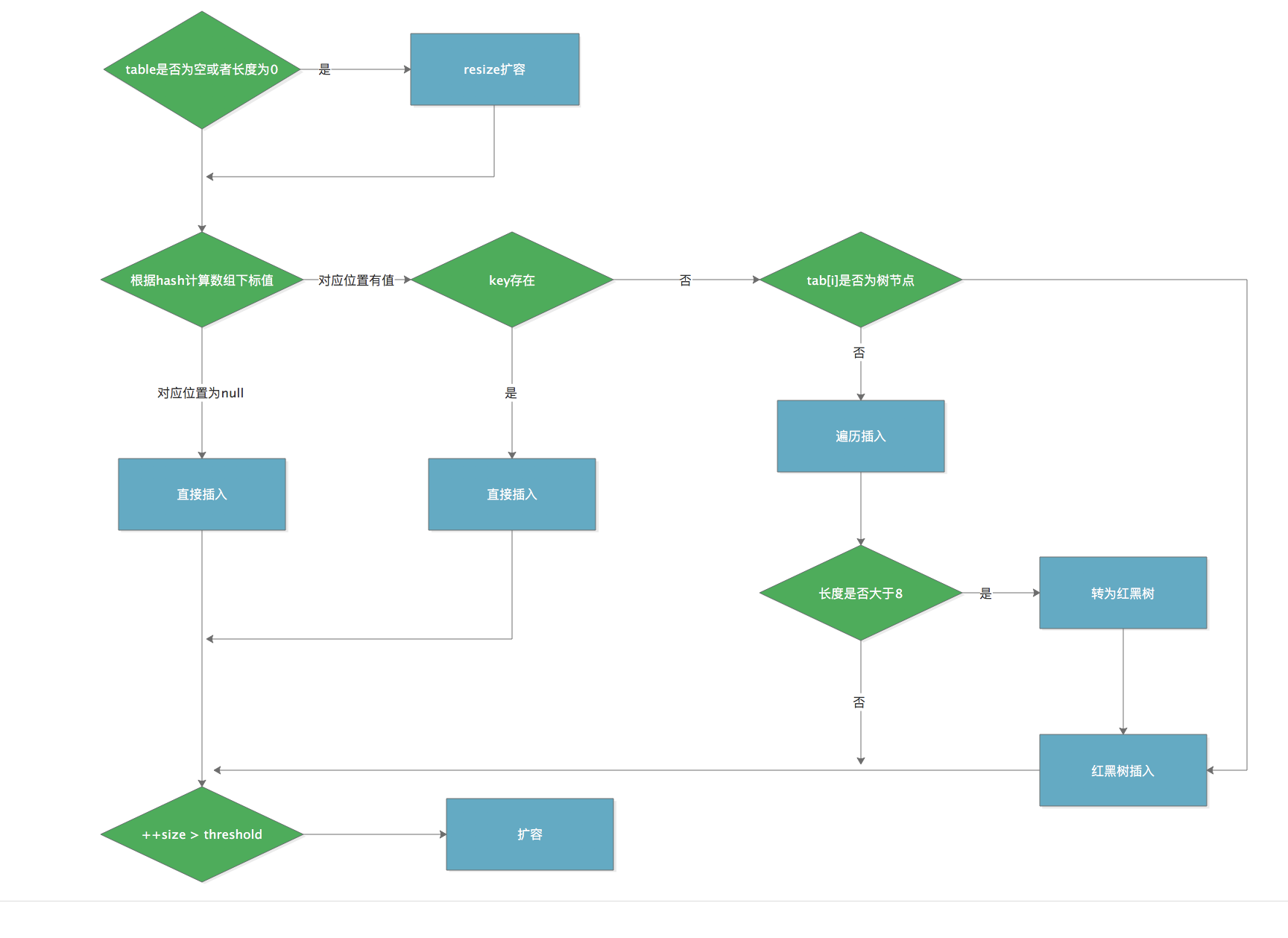get()方法

public V get(Object key) {
Node<K,V> e;
/** 首先将 key hash 之后取得所定位的桶 */
return (e = getNode(hash(key), key)) == null ? null : e.value;
}
final Node<K,V> getNode(int hash, Object key) {
Node<K,V>[] tab; Node<K,V> first, e; int n; K k;
/** 如果桶为空则直接返回 null  */
if ((tab = table) != null && (n = tab.length) > 0 &&
(first = tab[(n - 1) & hash]) != null) {
/** 否则判断桶的第一个位置(有可能是链表、红黑树)的 key 是否为查询的 key，是就直接返回 value */
if (first.hash == hash && // always check first node
((k = first.key) == key || (key != null && key.equals(k))))
return first;
/** 如果第一个不匹配，则判断它的下一个是红黑树还是链表 */
if ((e = first.next) != null) {
/** 红黑树就按照树的查找方式返回值 */
if (first instanceof TreeNode)
return ((TreeNode<K,V>)first).getTreeNode(hash, key);
/** 链表就遍历匹配返回值 */
do {
if (e.hash == hash &&
((k = e.key) == key || (key != null && key.equals(k))))
return e;
} while ((e = e.next) != null);
}
}
return null;
}

1.3.HashMap线程不安全的原因

resize造成死循环

Jdk1.7中transfer方法如下：

void transfer(Entry[] newTable, boolean rehash) {
int newCapacity = newTable.length;
for (Entry<K,V> e : table) {
while(null != e) {
Entry<K,V> next = e.next;
if (rehash) {
e.hash = null == e.key ? 0 : hash(e.key);
}
int i = indexFor(e.hash, newCapacity);
e.next = newTable[i];
newTable[i] = e;
e = next;
}
}
}

transfer方法的的作用就是：

• 对索引数组中的元素遍历
• 对链表上的每一个节点遍历：用 next 取得要转移那个元素的下一个，将 e 转移到新 Hash 表的头部，使用头插法插入节点。
• 循环2，直到链表节点全部转移
• 循环1，直到所有索引数组全部转移